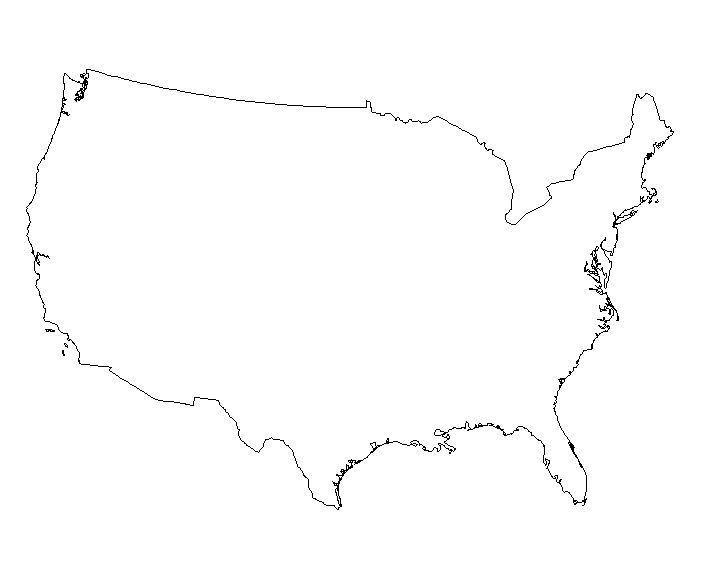# Rules for significant figures

 Date 28.03.2018 Size 36.75 Kb.

### RULES FOR SIGNIFICANT FIGURES

The Atlantic Pacific Rule
 (1) Pacific – "P" is for decimal point is present. If a decimal point is present, count significant digits starting with the first non-zero digit on the left.

 Examples: (a) 0.004703 has 4 significant digits. (b) 18.00 also has 4 significant digits.

 (2) Atlantic – "A" is for decimal point is absent. If there is no decimal point, start counting significant digits with the first non-zero digit on the right.

 Examples: (a) 140,000 has 2 significant digits. (b) 20060 has 4 significant digits.

 Imagine a map of the U.S.; If the decimal is absent count from the Atlantic side. If the decimal point is present, count from the Pacific side. In both cases, start counting with the first non-zero digit. PA

 Calculations shouldn't have more precision than the least precise measurement. This leads to 2 rules:

 (A) For addition and subtraction: The answer should not have more places past the decimal than the number with the least places past the decimal.

 Example: 1.2 + 12.348 = 13.5 Not 13.548

 (B) For multiplication and division: The answer should not have more significant figures than the number with the least amount of significant figures.

 Example: 502 x 3.6 = 1800 Not 1807.2

 * These last 2 rules can be called the Many-Places rule. For mult/div, how many significant figures is important. For plus/minus, number of places is important.